# Algebra 101: Beginner to Intermediate Level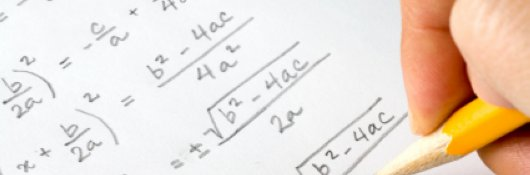\$85.00
no certificate
- OR -
\$110.00
with CEU Certificate*
Start Right Now!
Taking multiple courses? Save with our platinum program.

• 14
Lessons
• 24
Exams &
Assignments
• 5,991
Students
have taken this course
• 25
Hours
average time
• 2.5
CEUs

## Course Description

Beginner to Intermediate Algebra is intended for students who need to gather a basic understanding of how to perform Algebra operations. This course will benefit current students and adult learners who need to know how to perform basic Algebra operations which involve simplifying, factoring, or solving equations in the form of inequalities, fractions, exponents and word problems.

This online course takes the student's knowledge of arithmetic and extends it by introducing the concepts of variables and functions. These new concepts allow students to solve a range of problems involving unknown quantities in areas such as geometry, probability and statistics, and various real-world scenarios.

Even from their childhood days, most people have at least a basic grasp of arithmetic: addition and subtraction of numbers, for instance. As we master these basic operations, we begin to search for a more systematic and rigorous understanding of the behavior of numbers (particularly, sets of numbers) and functional relationships. This search is where we make the step from arithmetic to algebra. Algebra is the systematic study of numbers (or, more generally, quantity) and their relationships at an abstract level. By studying algebra, you can acquire the ability to analyze the relationships between numbers generally, rather than simply between particular numbers. These abilities pave the way for understanding other areas of mathematics such as trigonometry and calculus.

• Completely Online
• Self-Paced
• 6 Months to Complete
• Start Anytime
• PC & Mac Compatible
• Android & iOS Friendly
• Accredited CEUs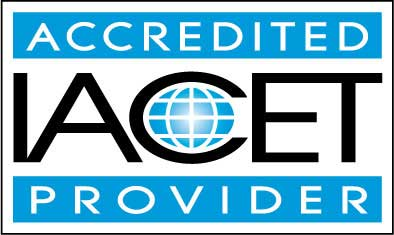## Course Lessons

Average Lesson Rating: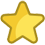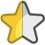#### Lesson 1: Algebra Terms and Motivation

This lesson will introduce you to the essential terminology of algebra as well as the reasons why studying algebra is important and helpful. 11 Total Points
•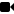Lesson 1 Video A
•Lesson 1 Video B : How to Take an Exam
•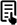Review 3 Articles: Graphs and Functions; Summary of Numbers Used in Algebra; Variables
•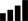Take Survey: Reasons for Taking this Course
•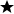Complete Assignment: Introduction
•Complete Exam: Lesson 1 – Algebra Terms and Motivation

#### Lesson 2: The Set of Real Numbers

Now that you have gained a grasp of the essential purpose of and some of the important terms used in algebra, we can move on to study the subject in more depth. 10 Total Points
•Lesson 2 Video
•Review Article: Real Numbers
•Complete Exam: Lesson 2 – The Set of Real Numbers

#### Lesson 3: Algebraic Operations

In this lesson, you will learn (or review) how to perform addition, subtraction, multiplication, and division in the context of algebra. 18 Total Points
•Lesson 3 Video
•Review Article: Algebraic Properties of Equality
•Complete: Lesson 3 Exercises
•Complete Exam: Lesson 3 – Algebraic Operations

#### Lesson 4: Functions

Once you have mastered the basic concepts associated with functions, you will then have all the tools you need to get into the more complicated (and more fun) aspects of algebra. 18 Total Points
•Lesson 4 Video
•Review Article: Composing functions
•Complete: Lesson 4 Exercises
•Complete Exam: Lesson 4 - Functions

#### Lesson 5: Equations, Linear Equations and Functions

This lesson will introduce you to equations, linear equations and functions, including their properties, graphs, and solutions. 19 Total Points
•Lesson 5 Video
•Complete: Lesson 5 Exercises
•Complete Exam: Lesson 5 – Linear Equations

#### Lesson 6: Linear Inequalities

A slight twist on the concept of a linear equation is the linear inequality. Linear inequalities, instead of equating two functions, relate two functions in terms of their relative magnitudes. 14 Total Points
•Lesson 6 Video
•Complete: Lesson 6 Exercises
•Complete Exam: Lesson 6 – Linear Inequalities

#### Lesson 7: Systems of Linear Equations

This lesson will teach you how to solve systems of linear equations using substitution and will extend your understanding beyond simple equations in two dimensions to equations in many dimensions. 10 Total Points
•Lesson 7 Video
•Complete Exam: Lesson 7 – Systems of Linear Equations

#### Lesson 8: Systems of Linear Inequalities

As with systems of linear equations, we can also handle systems of linear inequalities. 10 Total Points
•Lesson 8 Video
•Complete Exam: Lesson 8 – Systems of Linear Inequalities

#### Lesson 9: Solving Word Problems

This lesson, then, will teach you some basic skills for dealing with problems that can arise in the real world. 14 Total Points
•Lesson 9 Video
•Complete: Lesson 9 Exercises
•Complete Exam: Lesson 9 – Solving Word Problems I

#### Lesson 10: Polynomial Functions

This lesson, then, will teach you some basic skills for dealing with problems that can arise in the real world. 18 Total Points
•Lesson 10 Video
•Complete: Lesson 10 Exercises
•Complete Exam: Lesson 10 – Polynomial Functions

#### Lesson 11: Exponential and Logarithmic Functions

Now that we have covered polynomials, we will look at a special class of functions that differ quite drastically: logarithmic and exponential functions. 10 Total Points
•Lesson 11 Video
•Complete Exam: Lesson 11 – Exponential and Logarithmic Functions

#### Lesson 12: Polynomial Functions II

In this lesson, we will learn how to find solutions to higher-degree polynomial equations. 12 Total Points
•Lesson 12 Video
•Complete: Lesson 12 Exercises
•Complete Exam: Lesson 12 – Polynomial Functions II

#### Lesson 13: Application of Algebra to Geometry

In this lesson, we will apply what we've learned about algebra to the study of geometry. If you've carefully followed the course up to this point, much of what is discussed here should hold no surprises for you. 13 Total Points
•Lesson 13 Video
•Complete: Lesson 13 Exercises
•Complete Exam: Lesson 13 – Application of Algebra to Geometry

#### Lesson 14: Application of Algebra to Statistics and Probability

Although this lesson assumes some prior knowledge of probability and statistics, the concepts presented from these areas are relatively simple and understandable. 56 Total Points
•Lesson 14 Video
•Take Poll: Let us know what you think of this course
•Take Survey: Program Evaluation Follow-up Survey (End of Course)
•Complete Exam: Lesson 14 – Application of Algebra to Statistics and Probability
•Complete: The Final Exam
233
Total Course Points

## Learning Outcomes

By successfully completing this course, students will be able to:
• Identify and define Algebra terms.
• Identify Real Numbers.
• Perform basic Algebraic Operations.
• Identify and create Algebra Functions.
• Identify and solve Linear Equations.
• Identify and solve Linear Inequalities.
• Identify and solve Algebra Word Problems.
• Identify and solve Polynomial Functions.
• Identify and solve Exponential and Logarithmic Functions, and
• Demonstrate mastery of lesson content at levels of 70% or higher.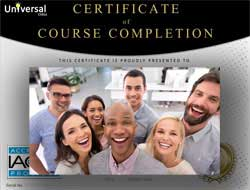• Document Your Lifelong Learning Achievements
• Earn an Official Certificate Documenting Course Hours and CEUs
• Verify Your Certificate with a Unique Serial Number OnlineCourse Title: Algebra 101: Beginner to Intermediate Level
Course Number: 9770451
Course Requirements: View Course Requirements
Lessons Rating: 4.3 / 5 Stars (1,225 votes)
Languages: English - United States, Canada and other English speaking countries
Category:
Course Type: General Education (Self-Paced, Online Class)
CEU Value: 2.5 IACET CEUs (Continuing Education Units)
CE Accreditation: Universal Class, Inc. has been accredited as an Authorized Provider by the International Association for Continuing Education and Training (IACET).
Assessment Method: Lesson assignments and review exams
Course Author: Dr. Jeffrey Clark, Ph.D.
Instructor: April Cordry-Moore
Syllabus: View Syllabus
Duration: Continuous: Enroll anytime!
Course Fee: \$85.00 (no CEU Certification) || with Online CEU Certification: \$110.00

Course Only
One Course
No Certificate / No CEUs
\$85
for 6 months

Billed once
This course only
 Includes certificate X Includes CEUs X Self-paced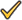Instructor supportTime to complete 6 months No. of courses 1 course
Certificate Course
One Course
Certificate & CEUs
\$110
for 6 months

Billed once
This course only
 Includes certificateIncludes CEUsSelf-pacedInstructor supportTime to complete 6 months No. of courses 1 course
Platinum Yearly
ALL COURSES
Certificates & CEUs
\$189
per year

Billed once
Includes all 500+ courses
 Includes certificateIncludes CEUsSelf-pacedInstructor supportTime to complete 12 Months No. of courses 500+
Platinum 2 Years
ALL COURSES
Certificates & CEUs
\$299
for 2 years
You save \$79.00!
Billed once
Includes all 500+ courses
 Includes certificateIncludes CEUsSelf-pacedInstructor supportTime to complete 24 Months No. of courses 500+

## Student Testimonials

• "I enjoyed being able to get in touch with the professor." -- Sarah B.
• "The instructor was very knowledgeable about Algebra and very helpful when I had questions." -- Donna N.
• "The lesson assignments were very helpful. The netlinks were very helpful too. This is great for CEU. It helped me work off the rust. Great!!!!" -- William J.
• "All parts were very helpful." -- Maliaka M.
• "He was really good! The teacher emailed me back so quick when I had a question and that helped more than anything else! He emailed me back so quick that I could just get on with my courses and not really have to stop." -- Claire S.
• "Prompt reply to my questions." -- Zeeshan M.